# AN127 - MA102/MA3XX - Rotor Direct Angle Sensing with a MagAlphaGet valuable resources straight to your inbox - sent out once per month

## ABSTRACT

The MagAlpha can detect the absolute rotor position of a brushless motor in real time, even without a target magnet, by measuring the fringe field of the rotor. The sensor must be mounted at the correct place to get the maximum rotor magnetic field without being disturbed by other fields, particularly the windings field. For this application note, a commercially available BLDC motor with three Hall switches is used as an example. The rotor magnetic field was measured and an adequate positon was determined from this information. By simply replacing the three Hall switches with the MagAlpha, the BLDC motor can be driven with more efficient schemes than block commutation (trapezoidal, sine drive, field oriented control) or used for speed or position control (see Figure 1).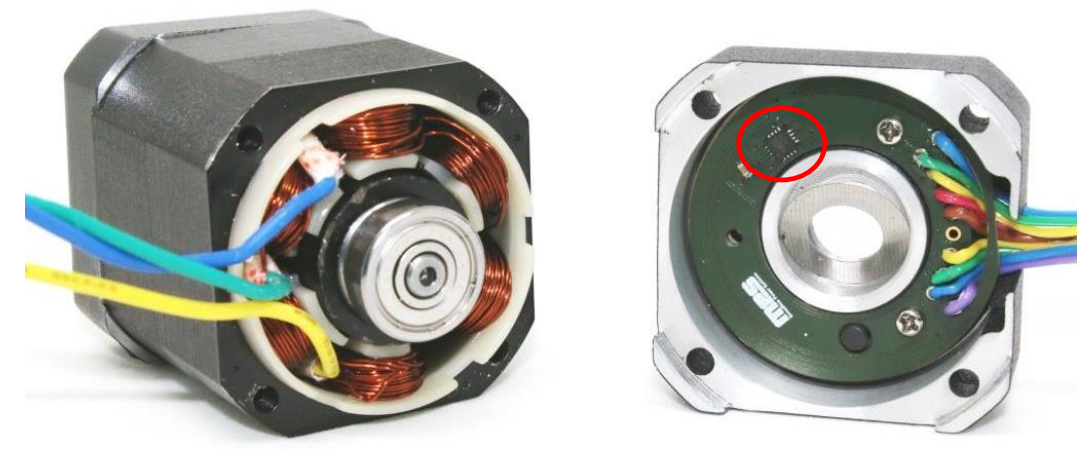## INTRODUCTION

The sensor replacement was done in a 4-pole pair BLDC motor with a rated speed of 4000 rpm (see Table 1 and Figure 2).

 Parameter Unit Value Number of poles pairs 4 Number of phase 3 Rated voltage V 24 Rated phase current A 3.5 Rated speed rpm 4000 Torque constant Nm/A 0.04 Line to line inductance mH 1.2

Table 1: BLDC Motor Specifications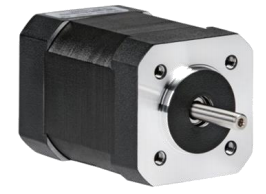Figure 2: 4-Pole Pair BLDC Motor

Originally, the three Hall switches were mounted on a PCB close to one extremity of the rotor. To upgrade the motor with minimum changes, the new PCB (with the MagAlpha) must be mounted at the exact same position as the PCB holding the three Hall switches (see Figure 3). Because of this constraint, the MagAlpha center is 1.5mm above the rotor magnet in the axial direction. The optimum radial position of the MagAlpha still needs to be determined. For block commutation, only the I/O of the new PCB are the same as the original one, except for the supply voltage (see Figure 4). For more features requiring the absolute position, extra leads to the PCB are required (see Figure 5).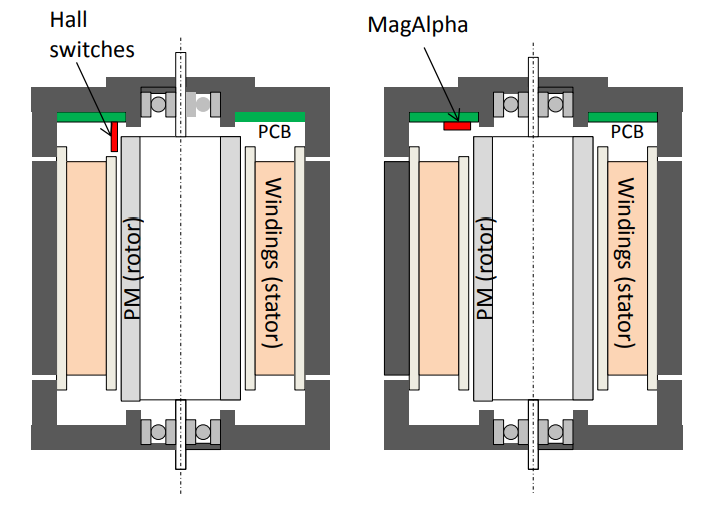Left: with Three Hall Switches.

Right: with the MagAlpha

Figure 3: Cross Section of the BLDC Motor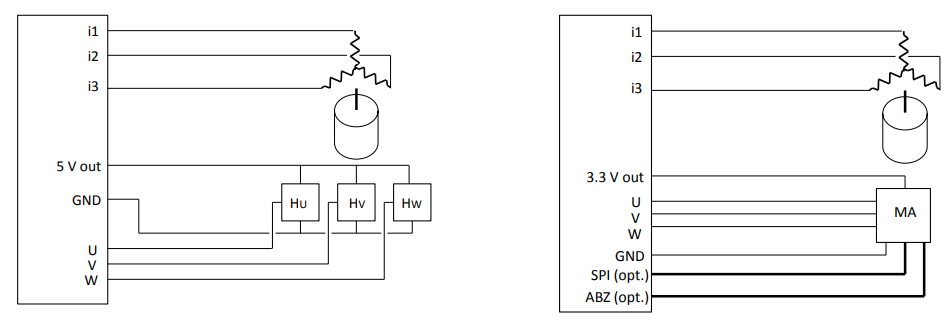Left: with Three Hall Switches.

Right: with the MagAlpha

Figure 4: Motor Control Schematic

During the tests, the motor was driven by a Maxon drive board DEC module 24/2. The MagAlpha used was an MA302, but the results apply for any MA sensor.

## ROTOR MAGNETIC FIELD

### Measurement Setup

The magnetic field produced by the rotor is examined with a 3D probe (MagVector from Metrolab) by scanning along the radius of possible positions. For each radius, the rotor is turned over a full revolution. The rotor angle is controlled with a precise (±0.001 deg) rotator.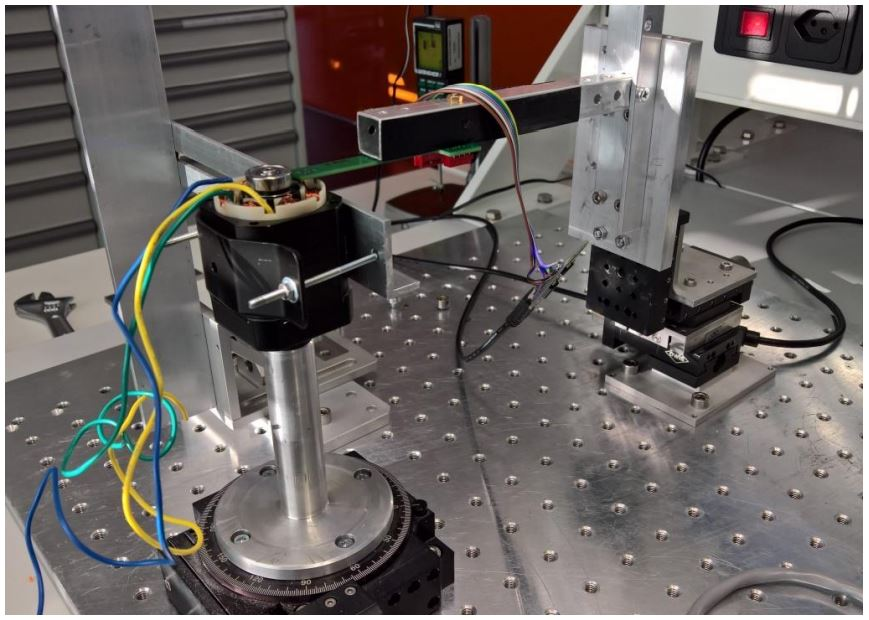Figure 5: Experimental Setup for Measuring the Three Components of the Rotor Magnetic Field

### Field of the Naked Rotor

The rotor is inserted in the rotator, and the 3D probe is scanned maintaining a vertical distance of 1.5mm above the rotor (see Figure 6). The three components of the rotor field are sinusoidal (see Figure 7). Bt, Br, and Bz are the amplitude of the tangential, radial, and axial components respectively. The MagAlpha senses only the radial and tangential component. Therefore, the first criterion is that Br and Bt must be sufficiently large. Figure 8 shows these amplitudes along the radius (where r is the rotor outer radius). At r = 0, the sensor center is just above the rotor outer radius.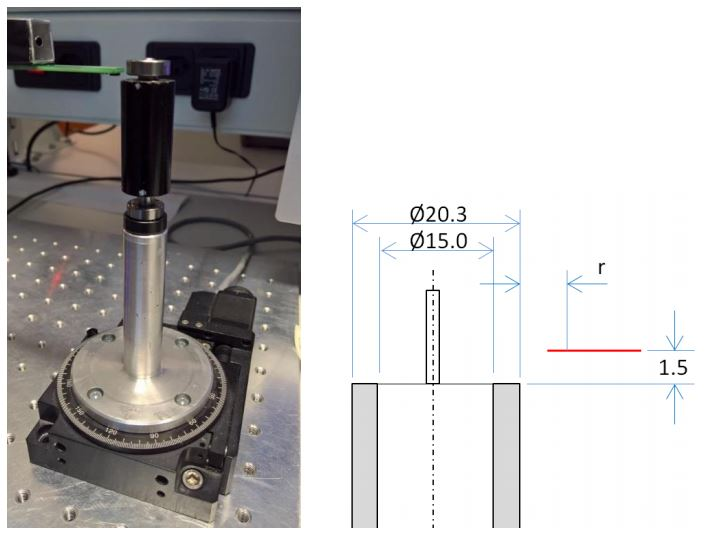Figure 6: Setup Used to Scan the Magnetic Field of the Naked Rotor (Red Line: Scan Path)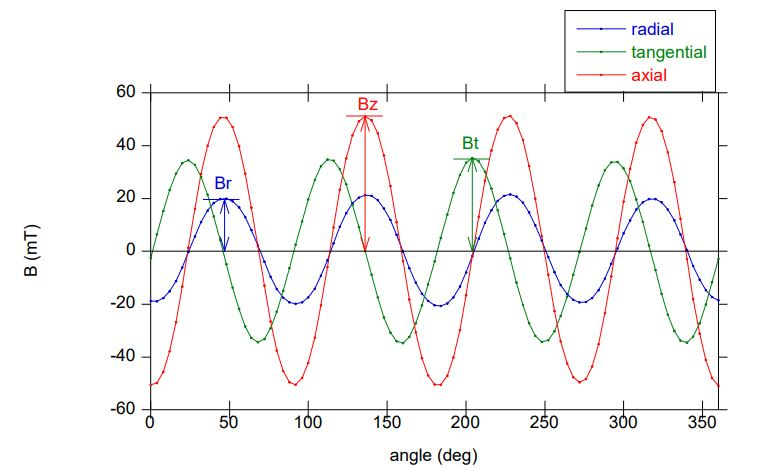Figure 7: 3D Field Measurement at the Position r = 1.5mm and z = 1.5mm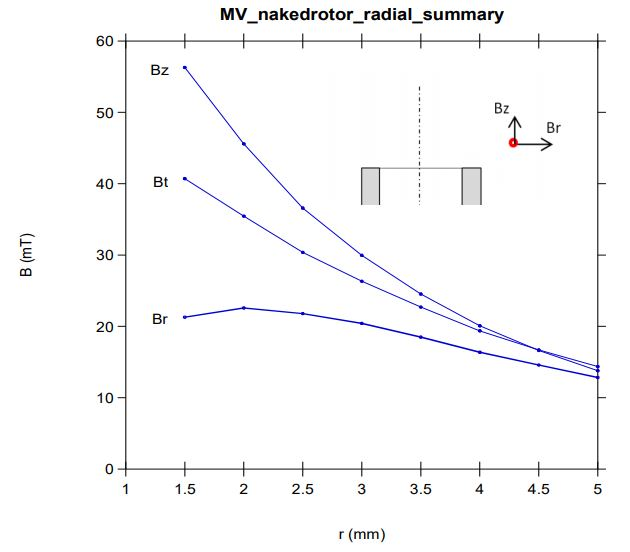Figure 8: Amplitude of the Three Components of the Magnetic Field along a Radius (z = 1.5mm)

### Field of the Rotor + Stator

In real conditions, the MagAlpha can also sense the effect of the iron core of the stator, which is expected to modify the field of the rotor. To determine exactly how much, a 3D scan was performed with an immobile stator as the rotor was rotated. This the motor casing is clamped as shown in Figure 9.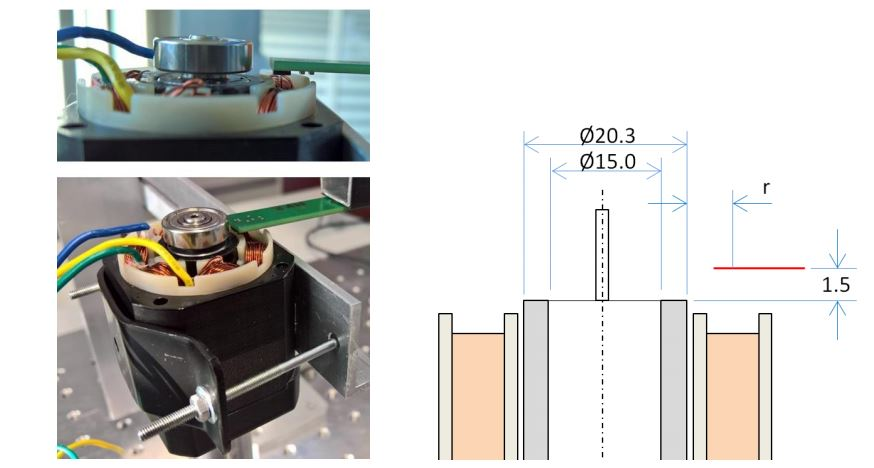Figure 9: Setup for Measuring the Rotor Field in Real Motor Environment (i.e.: with the Stator)

Figure 10 shows the results. Note that the windings are not energized. Noticeably, the radial magnetic field is not modified by the presence of the stator, while the tangential field is reduced significantly. This reduction means that the stator provides a return path for the tangential field. Consequently, the field strength at a radius larger than 3mm is too low. When the radius is about 2.5mm, the magnetic field is near optimum with Br and Bt being almost equal.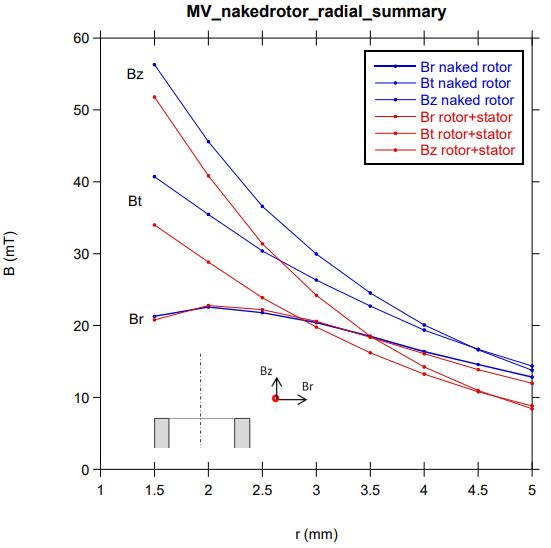Figure 10: Amplitude of the Three Components of the Magnetic Field along a Radius (z = 1.5mm)

Another criterion for finding the optimum position is the sensor linearity. This means that after the MagAlpha on-chip linearization (BCT adjustment), the non-linearity should be as small as possible. The on-chip linearization can compensate for elliptic errors (i.e.: errors caused by Bt being different from Br). However, if the radial and tangential components are not perfectly sinusoidal, there is some residual nonlinearity, which cannot be compensated by the BCT adjustment. Figure 11 shows the first and second harmonic residual errors (H1 and H2) resulting from the field distortion as a function of the sensor position. These errors increase if the radius is smaller than 2.5mm. Note that the total INL is larger than H1 + H2 (around four deg). This error is due to the non-equal distance between the rotor poles (see Figure 12).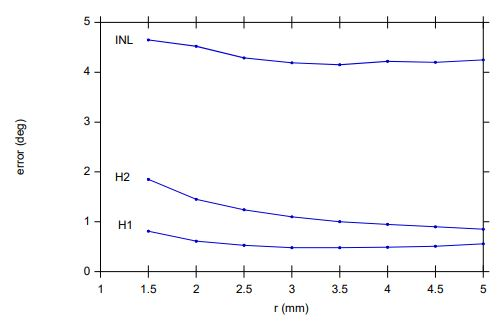Figure 11: Residual Non-Linearity Due to the Rotor Field Distortion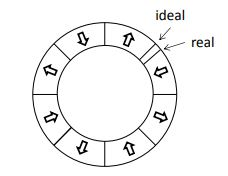Figure 12: Non-Ideal Rotor Magnet

The field strength and the linearity criteria together suggest that a radius of 2.5mm provides a reasonable tradeoff (see Figure 13). This position is close to the maximum radial field (Br) of the naked rotor. Therefore, as a rule of thumb, the radius for maximum radial field of a naked rotor can be used as the MagAlpha position.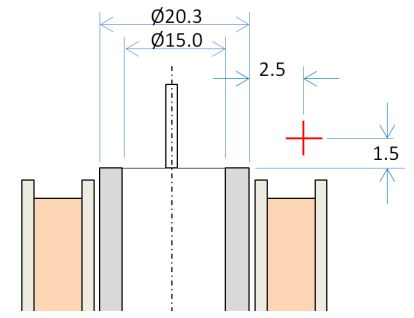Figure 13: Optimum Position for the MagAlpha

From the magnetic field data, it is possible to calculate how much error increase is caused by the magnetic configuration (see Figure 14). The eighth harmonics comes from the difference between Bt and Br within each pole pair and by the non-perfect sinusoidal field. This error can be compensated partially in the MagAlpha by adjusting the BCT parameter. The first harmonic error comes from the rotor’s imperfect pole sizes. Note that the scale is in magnetic degrees. In mechanical degrees, the overall nonlinearity is around one deg.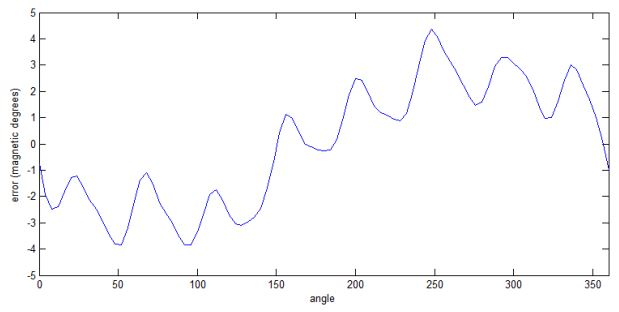Figure 14: Error Curve at r = 2.5mm due to the Non-Ideal Rotor Magnet in Magnetic Degrees (Absolute Angle Error (i.e.: Mechanical Degrees) is 4x Smaller)

### Effect of the Winding Current

When the motor is running, some stray fields are also generated by the winding current. A DC current flowing into two of the three leads creates a tangential field at the MagAlpha position. For the motor used here, at the selected position (r = 2.5mm), a current of 1.5A shifts the tangential field by about 2mT (see Figure 15). On the other hand, the radial component remains unaffected by the winding current. Consequently, the winding current creates a small rotation (3.8 deg at 1.5A) of the magnetic vector measured by the MagAlpha. Figure 16 shows this rotation as a function of the rotor angle. Since the winding current effect is linear (and makes 2.5 magnetic deg/A), the angle rotation is about nine magnetic degrees at the motor rated phase current (3.5A). Note that this error does not affect the motor operation significantly when the MagAlpha signals are used for commutation.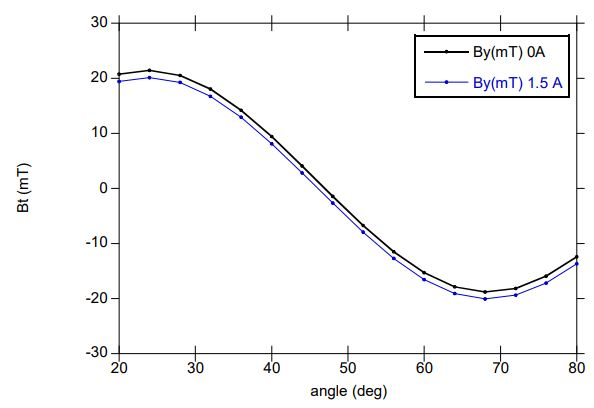Figure 15: Effect of 1.5A Static Winding Current on the Tangential Magnetic Field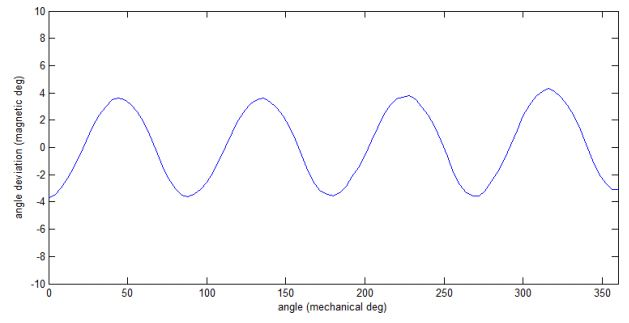Figure 16: Effect of 1.5A Static Current on the Measured Angle

## SETTINGS OF THE MAGALPHA AND RESULTS

Figure 17 shows the recommended PCB four mounting the MagAlpha in accordance to the best position point (r = 2.5 mm).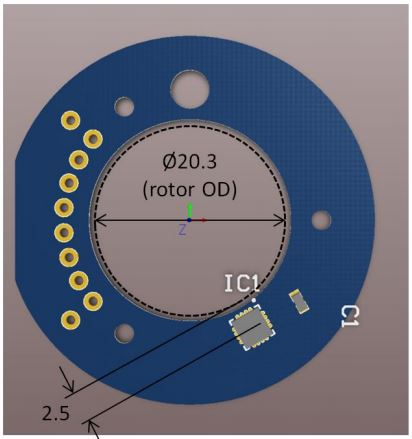Figure 17: New PCB

### Zero Setting

At zero degrees, the MagAlpha raises the edge of its U channel. Therefore, the MagAlpha zero angle must coincide with the raising edge of the U Hall switch. In the motor used here, this transition occurs when the rotor is free to move and the phase current is applied between i2 and i3 (see Figure 18).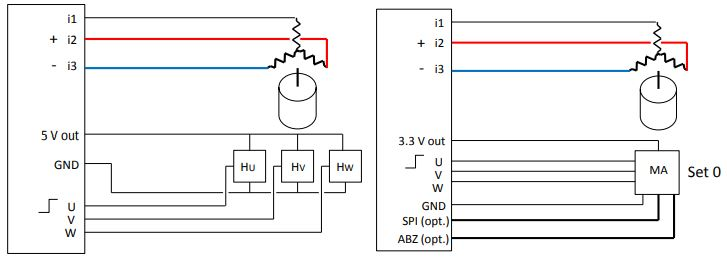Figure 18: Zero Position Setting by Energizing the Windings

The zero is set using the SPI communication board of the evaluation kit (MACOM) and user interface (MACOM app). Once the zero is set, the motor runs smoothly. The same PCB was tested in two similar motors. Figure 19 shows the sensor SPI output while the motor is rotating at 20krpm driven by the MagAlpha UVW output. Because of the motor inertia, the rotation speed is assumed to be almost constant, and therefore the displayed error curve and INL represent the system non-linearity. Since the error curve shows eight oscillations, the non-linearity is dominated by a second harmonic of about 2.3 deg in each magnetic revolution (which is an eighth harmonic if considering one rotor mechanical revolution) as expected from the 3D probe measurements (see Figure 14). This is mainly due to the distortion of the rotor field from a perfect sinewave. Note that the MagAlpha intrinsic non-linearity also contributes to this error. Since the MagAlpha position is close to the point where Br = Bt, little or no BCT adjustment is required. With the two motors tested, it was found that for one of them, the INL could be reduced by 0.2 deg by setting BCT = 12. For the other motor, the best INL was obtained with the default BCT setting (i.e.: BCT = 0).

The effect of the non-ideal pole periodicity (causing an INL of ±4 deg, see Figure 14) is not visible on the error curve. It is suggest that the motor speed varies slightly (about 1 %) during one rotation. This variation should also occur with the three Hall switches. Table 2 shows the accuracy performances. Note that since the perfomance is independent of the rotation speed, a calibration curve can also be stored in an external device for precise position control. A flywheel can also be added for guaranteeing a constant roation speed during calibration.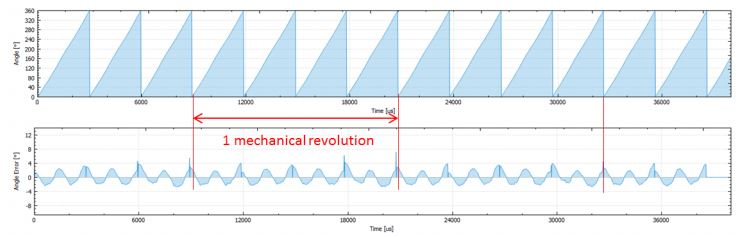Top: Raw Output

Bottom: Error Curve (Difference between the Raw Output and a Constant Speed Curve)

Figure 19: MagAlpha SPI Output Measured with the MACOM App while the Motor Turns at 20krpm

 Motor 1 (BCT = 12) Motor 2 (BTC = 0) Parameter Magnetic Deg Mechanical Deg Magnetic Deg Mechanical Deg INL 2.7 0.7 3.0 0.75 H1 0.5 1.13 0.13 0.22 H2 2.3 0.57 0.57 0.55

Table 2: Sensor Accuracy Performance

## SUMMARY: STEP-BY-STEP PROCEDURE

Follow the below guidelines for mounting the MagAlpha for sensing the rotor angle without an additional magnet.

### 1. Choose a MagAlpha

Use Table 3 to select a MagAlpha device based on your needs.

 Purpose Device For block commutation only MA102 For block commutation + another output (SPI, ABZ) MA302 For block commutation + another output (SPI, ABZ) and high dynamics MA304

### 2. Find the Optimum Radius for Placing the MagAlpha (Maximum BR)

The most accurate way to measure the field is to use a 3D Hall sensor. The MagVector from Metrolab (MV2 magnetometer at www.metrolab.com) has the same form factor as the MagAlpha. Another option is to use the MagAlpha itself (see Figure 20).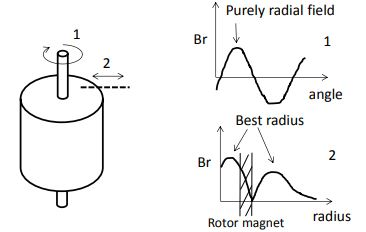Figure 20: Sketch of the Radial Magnetic Field (Br) Produced by a Rotor

(Top Graph: Br along an Arc, Bottom Graph: Br along a Radius)

Take the rotor apart and mount it with a bearing. Rotate it until the 3D magnetic sensor shows the maximum of the radial component. At this angle, the tangential component should be zero. Then move the magnetic sensor radially (ideally with a micrometer stage) until a maximum is detected. Alternatively, the optimum radius can be found using the MagAlpha as a gaussmeter. For this, two-way SPI communication is necessary. The MACOM evaluation kit can be used. Rotate the rotor until the MagAlpha angle output indicates a purely radial field. This depends on how the MagAlpha is oriented (see Figure 21).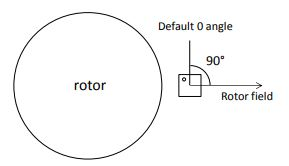Figure 21: Purely Radial Field Corresponding to a Reading of 90 Deg

Move the MagAlpha radially and measure the field strength.

• Coarse Detection: Adjust the MGL threshold to be one increment below the transition. This provides a field within 15mT.
• Fine Tuning: Decrease the Hall bias current (by enabling both ETX and ETY and increasing BCT) until the MGL flags is set. The BCT value is the fine scale value

As a cross-check, the same procedure can be used for the tangential field. At the optimum point, the k ratio (equal to Br/Bt) should be close to one. If this is not the case, a BCT setting is recommended for optimum results.

### 3. PCB Design and Fabrication

Center the PCB at the optimum radial position. Add a decoupling capacitor in accordance with the datasheet’s recommendations (see Figure 22).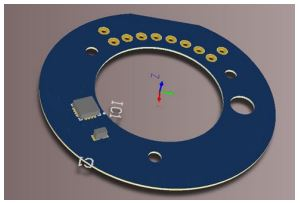Figure 22: Example of PCB with a MagAlpha and a Decoupling Capacitor

### 4. Set the Zero

If the motor was initially equipped with Hall switches, determine which winding bias turns the rotor close to the U channel rising edge. The configuration is the zero angle winding bias. Replace the old PCB with the new one, set the zero angle winding bias, and set the current position to zero (by SPI). The setting can be done easily with the MACOM interface (see Figure 23).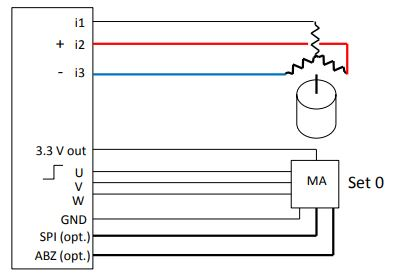Figure 23: Winding Supply Current for Zero Setting

Alternatively, if the motor was not initially equipped with Hall sensors, find the zero setting by observing which setting minimizes the motor current consumption at a fixed rpm. The motor is now ready to be used with the MagAlpha.